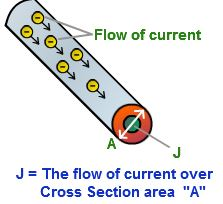Physics

# What is Current Density?

The current density at a point is defined as the quantity of charge passing per unit time through unit area, taken perpendicular to the direction of flow of charge at that point.The current density J for a current I flowing across a conductor having an area of cross section A is:

J = (q/t)/A = I/A

Current density is a vector quantity. It is expressed in A m-2